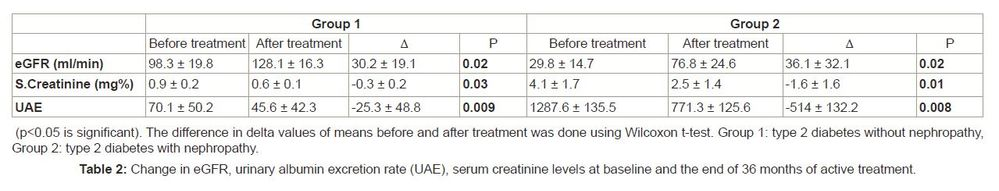## How to compute the percentage changes of continuous variables?

I have a phenotype data (contineous) from a group of patinets who treated a with a drug A. I would like to see what is the percentage inceremnet or decreamnet in the values of phenotype in those patinets. How can I do this?

I would like to plot the percentage changes in these variables!

7 REPLIES 7

## Re: How to compute the percentage changes of continuous variables?

Here is the reference paper: http://www.ncbi.nlm.nih.gov/pubmed/23260854

## Re: How to compute the percentage changes of continuous variables?

Post sample data and expected output. A sample of the graph your after is helpful, that article is behind a paywall.

A data step and proc SGPLOT are possibly all you require.

## Re: How to compute the percentage changes of continuous variables?

Here is the table of values and the plot which I want to make!## Re: How to compute the percentage changes of continuous variables?

Please post sample data in a useable format.

Ie I'm not typing out your data from a picture. Someone else may ...

## Re: How to compute the percentage changes of continuous variables?

Hi Thanks again! I have two columns here - one is the initial measurements and another is final measurement or after the treatment! Thats A_first and A_LastKsharp
Super User

## Re: How to compute the percentage changes of continuous variables?

```Give you an example:

data have;
input cat \$ group \$ res low high star;
cards;
F 1 12 10 14 16
F 2 14 12 15 .
M 1 20 18 22 23
M 2 18 17 20 .
;
run;
proc sgplot data=have;
vbarparm category=cat response=res
/group=group groupdisplay=cluster limitlower=low limitupper=high;
scatter x=cat y=star/discreteoffset=-0.22 markerattrs=(symbol=starfilled);
run;

proc sgplot data=have;
vbarparm category=cat response=res
/group=group groupdisplay=cluster limitlower=low limitupper=high datalabel;
scatter x=cat y=star/datalabel discreteoffset=-0.22 markerattrs=(symbol=starfilled);
run;

```

## Re: How to compute the percentage changes of continuous variables?

Thank you very much and let try this!
Discussion stats
• 7 replies
• 1362 views
• 1 like
• 3 in conversation